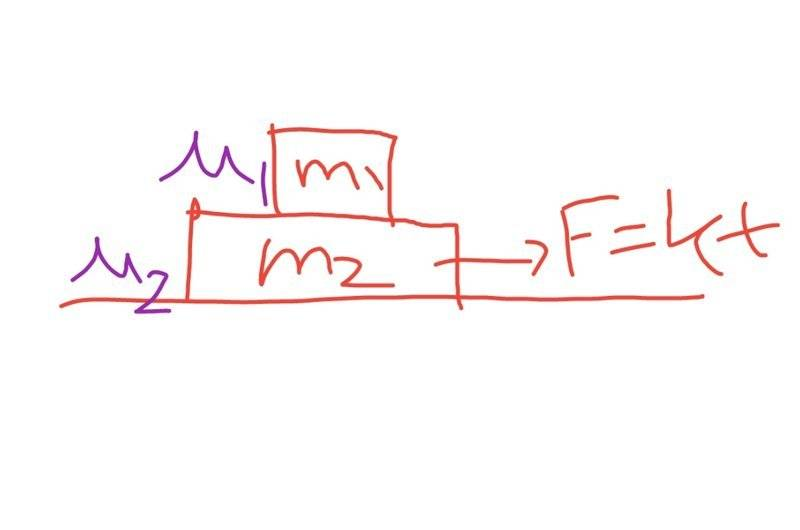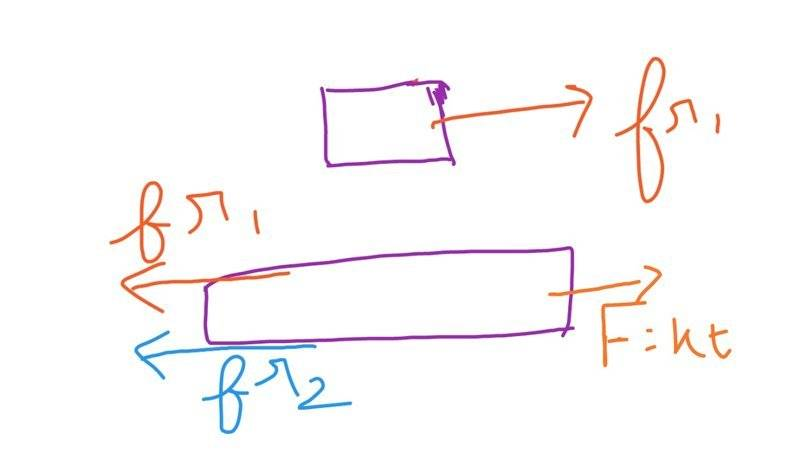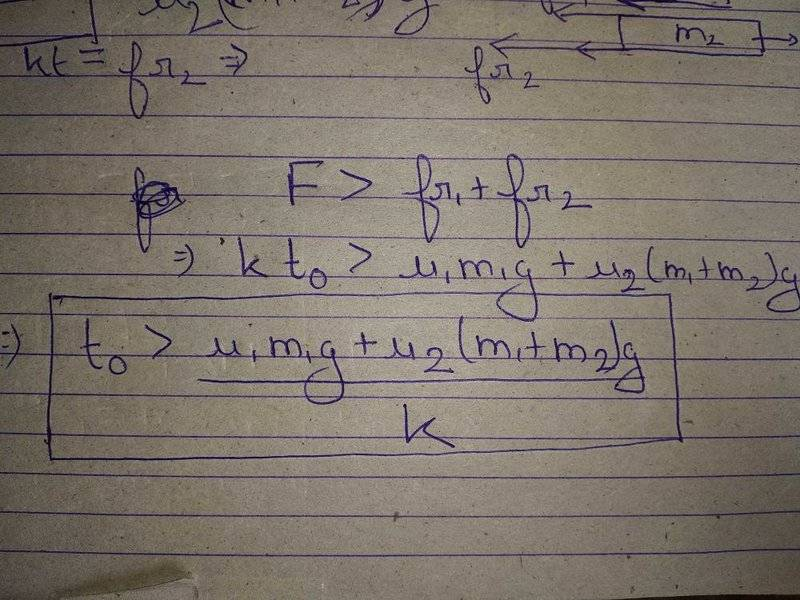# When Friction the block m2 start to move?

• navneet9431
In summary, the conversation discusses a scenario where a block m2 is placed on a surface with coefficient of friction μ2 and another block m1 is placed on top with coefficient of friction μ1. A force F=k*t is acting on block m2 and the question is when will block m2 start moving. The correct result is that the block m2 will start moving just after time "t" when the external force is greater than the sum of the frictional forces acting on block m2. This is because the maximum static friction is equal to μs*Normal force, but the static friction can take any value between zero and this maximum value.f

Gold Member

## Homework StatementA block m2 is placed on a surface where the coefficient of friction is μ2 and another block m1 placed over the block m2 where the coefficient of frictio is μ1.
A force F=k*t(Force as a function of time),is acting on the block m2.

Now my question is that when would the block m2 start moving?

## Homework Equations

F=ma

Static Friction=Coefficient Of Friction*Normal Force

## The Attempt at a Solution

I tried solving this by drawing the FBD of the objects.See two forces fr1 and fr2 will be acting on the block m2.
So m2 would start to move when F>fr1+fr2.
Or simply we can say k*t=fr1+ft2.
So the block m2 would start to move just after time "t".
Is my result correct?

#### Attachments

•Delta2
Yes it is, just be careful on how you going to equate forces fr1 and fr2 with relation to the weights ##m_1g ## and ##m_2g ## and the static friction coefficients ##\mu_1## and ##\mu_2##.

•navneet9431
Yes it is, just be careful on how you going to equate forces fr1 and fr2 with relation to the weights ##m_1g ## and ##m_2g ## and the static friction coefficients ##\mu_1## and ##\mu_2##.
So my result was correct.
See the pic I have uploaded.
Have I proceeded correctly?#### Attachments

Yes your result seems correct to me. Actually a minor correction, it should be ##t_0\geq…## not just >.

Last edited:
Static Friction=Coefficient Of Friction*Normal Force
There is a common blunder in that equation, and it is causing you to get the wrong answer.
For kinetic friction it works: ##F_k=\mu_kF_N##, but for static friction there is a key difference.

There is a common blunder in that equation, and it is causing you to get the wrong answer.
For kinetic friction it works: ##F_k=\mu_kF_N##, but for static friction there is a key difference.
You mean to say that my result is wrong?

You mean to say that my result is wrong?
Yes.

Yes.
And,also mention the correct result!

And,also mention the correct result!
Consider the situation when the applied force is zero. What are the frictional forces?

Zero
Consider the situation when the applied force is zero. What are the frictional forces?

Zero
Right, so what is wrong with ##F_s=\mu_sF_N##?

Ah, I think @haruspex is right, it is the maximum static friction that is equal to ##\mu_sF_N##. Static friction can take any value between zero and this maximum value.

When the box ##m_2## is about to move then the static friction between ##m_2## and the surface is indeed as you calculate ##fr_2=\mu_2(m_1+m_2)g## but box ##m_1## doesn't move with respect to box ##m_2## so the kinetic friction between m1 and m2 is just zero and also the static friction ##fr_1## is zero at the start of movement, later it gets bigger as the external force gets bigger.

Last edited: